Question

# A) A 25.7 mL sample of 0.264 M methylamine, CH3NH2, is titrated with 0.377 M hydrobromic...

A) A 25.7 mL sample of 0.264 M methylamine, CH3NH2, is titrated with 0.377 M hydrobromic acid. The pH before the addition of any hydrobromic acid is: ___

B) A 22.8 mL sample of 0.392 M dimethylamine, (CH3)2NH, is titrated with 0.378 M perchloric acid.
At the titration midpoint, the pH is: ___

(A) pH = 12.0

(B) pH = 10.8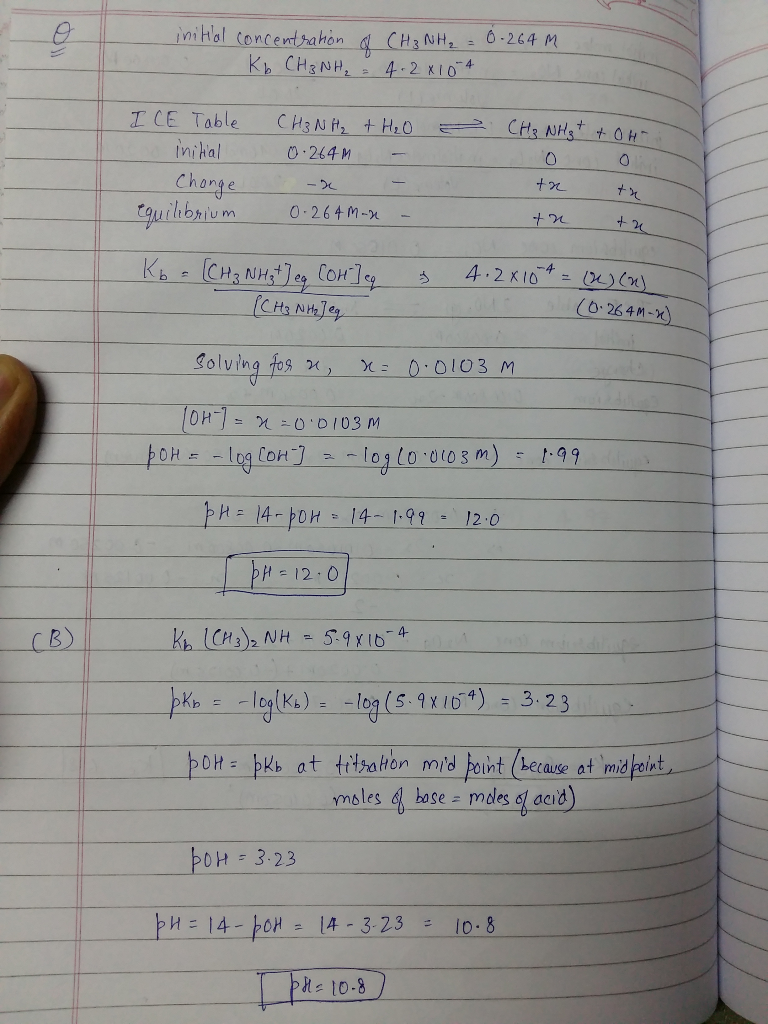#### Earn Coins

Coins can be redeemed for fabulous gifts.

Similar Homework Help Questions
• ### A 27.9 mL sample of 0.367 M methylamine, CH3NH2, is titrated with 0.260 M hydrobromic acid....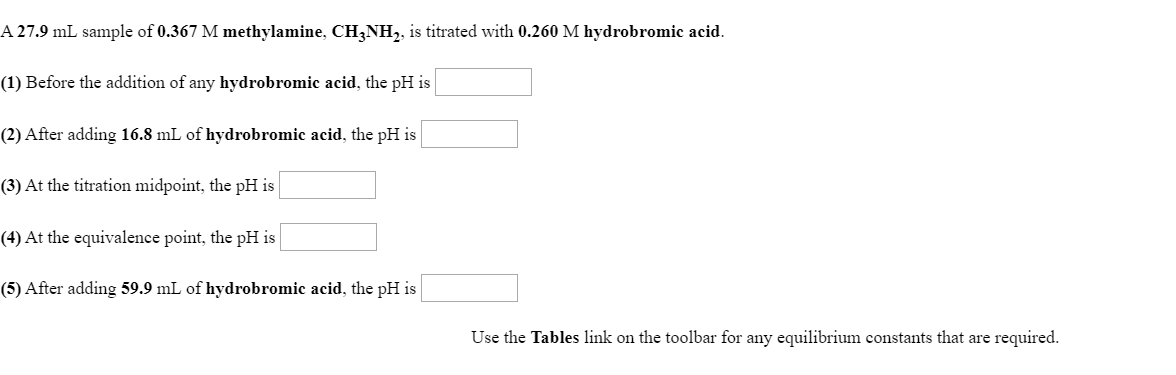A 27.9 mL sample of 0.367 M methylamine, CH3NH2, is titrated with 0.260 M hydrobromic acid. (1) Before the addition of any hydrobromic acid, the pH is (2) After adding 16.8 mL of hydrobromic acid, the pH is (3) At the titration midpoint, the pH is (4) At the equivalence point, the pH is (5) After adding 59.9 mL of hydrobromic acid, the pH is Use the Tables link on the toolbar for any equilibrium constants that are required.

• ### A 27.9 mL sample of 0.289 M dimethylamine, (CH3)2NH, is titrated with 0.286 M hydrobromic acid. ...

A 27.9 mL sample of 0.289 M dimethylamine, (CH3)2NH, is titrated with 0.286 M hydrobromic acid. (1) Before the addition of any hydrobromic acid, the pH is (2) After adding 12.0 mL of hydrobromic acid, the pH is (3) At the titration midpoint, the pH is (4) At the equivalence point, the pH is (5) After adding 45.1 mL of hydrobromic acid, the pH is Use the Tables link on the toolbar for any equilibrium constants that are required.

• ### A 21.5 mL sample of 0.367 M dimethylamine, (CH3)2NH, is titrated with 0.269 M hydroiodic acid....

A 21.5 mL sample of 0.367 M dimethylamine, (CH3)2NH, is titrated with 0.269 M hydroiodic acid. (1) Before the addition of any hydroiodic acid, the pH is (2) After adding 12.5 mL of hydroiodic acid, the pH is (3) At the titration midpoint, the pH is (4) At the equivalence point, the pH is (5) After adding 46.1 mL of hydroiodic acid, the pH is Ka of (CH3)2NH is 5.9x10^-4

• ### Ka of dimethylamine = 5.9*10^-4 A 21.5 mL sample of 0.383 M dimethylamine, (CH32NH, is titrated...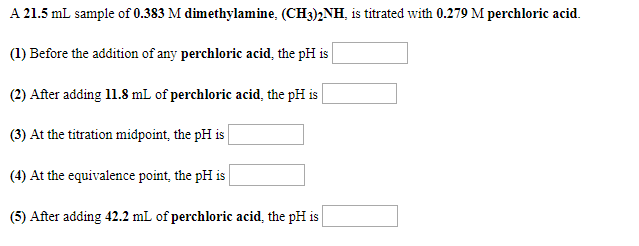Ka of dimethylamine = 5.9*10^-4 A 21.5 mL sample of 0.383 M dimethylamine, (CH32NH, is titrated with 0.279 M perchloric acid. (1) Before the addition of any perchloric acid, the pH is (2) After adding 11.8 mL of perchloric acid, the pH is (3) At the titration midpoint, the pH is (4) At the equivalence point, the pH is (5) After adding 42.2 mL of perchloric acid, the pH is

• ### A 29.5 mL sample of 0.300 M dimethylamine, (CH3)2NH, is titrated with 0.267 M hydrobromic acid....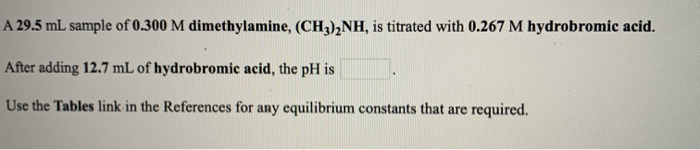A 29.5 mL sample of 0.300 M dimethylamine, (CH3)2NH, is titrated with 0.267 M hydrobromic acid. After adding 12.7 mL of hydrobromic acid, the pH is Use the Tables link in the References for any equilibrium constants that are required.

• ### A) A 27.5 mL sample of 0.223 M ethylamine, C2H5NH2, is titrated with 0.301 M hydrobromic...

A) A 27.5 mL sample of 0.223 M ethylamine, C2H5NH2, is titrated with 0.301 M hydrobromic acid. After adding 29.5 mL of hydrobromic acid, the pH is . B)  A 24.5 mL sample of 0.351 M diethylamine, (C2H5)2NH, is titrated with 0.302 M hydroiodic acid. At the titration midpoint, the pH is

• ### A 22.8 mL sample of 0.218 M methylamine, CH3NH2, is titrated with 0.393 M hydrochloric acid....

A 22.8 mL sample of 0.218 M methylamine, CH3NH2, is titrated with 0.393 M hydrochloric acid. After adding 4.64 mL of hydrochloric acid, the pH is .

• ### A 27.0 mL sample of 0.356 M ammonia, NH3, is titrated with 0.249 M hydrobromic acid....

A 27.0 mL sample of 0.356 M ammonia, NH3, is titrated with 0.249 M hydrobromic acid. (1) Before the addition of any hydrobromic acid, the pH is (2) After adding 16.8 mL of hydrobromic acid, the pH is (3) At the titration midpoint, the pH is (4) At the equivalence point, the pH is (5) After adding 55.6 mL of hydrobromic acid, the pH is

• ### A 27.2 mL sample of 0.270 M diethylamine, (C,Hz),NH, is titrated with 0.217 M hydroiodic acid....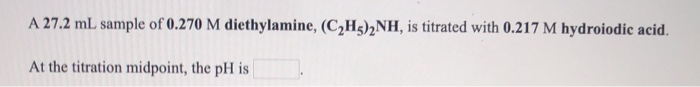A 27.2 mL sample of 0.270 M diethylamine, (C,Hz),NH, is titrated with 0.217 M hydroiodic acid. At the titration midpoint, the pH is A 22.6 mL sample of 0.215 M diethylamine, (C2H3)2NH, is titrated with 0.377 M hydroiodic acid. The pH before the addition of any hydroiodic acid is

• ### A 29.9 mL sample of 0.363 M triethylamine, (C2H3)3N, is titrated with 0.307 M nitric acid....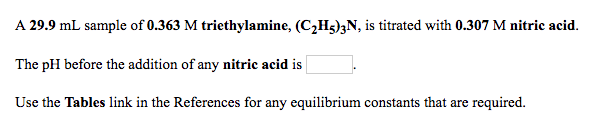A 29.9 mL sample of 0.363 M triethylamine, (C2H3)3N, is titrated with 0.307 M nitric acid. The pH before the addition of any nitric acid is Use the Tables link in the References for any equilibrium constants that are required. A 23.7 mL sample of 0.252 M diethylamine, (C2H3)2NH, is titrated with 0.349 M hydrobromic acid. At the titration midpoint, the pH is Use the Tables link in the References for any equilibrium constants that are required.